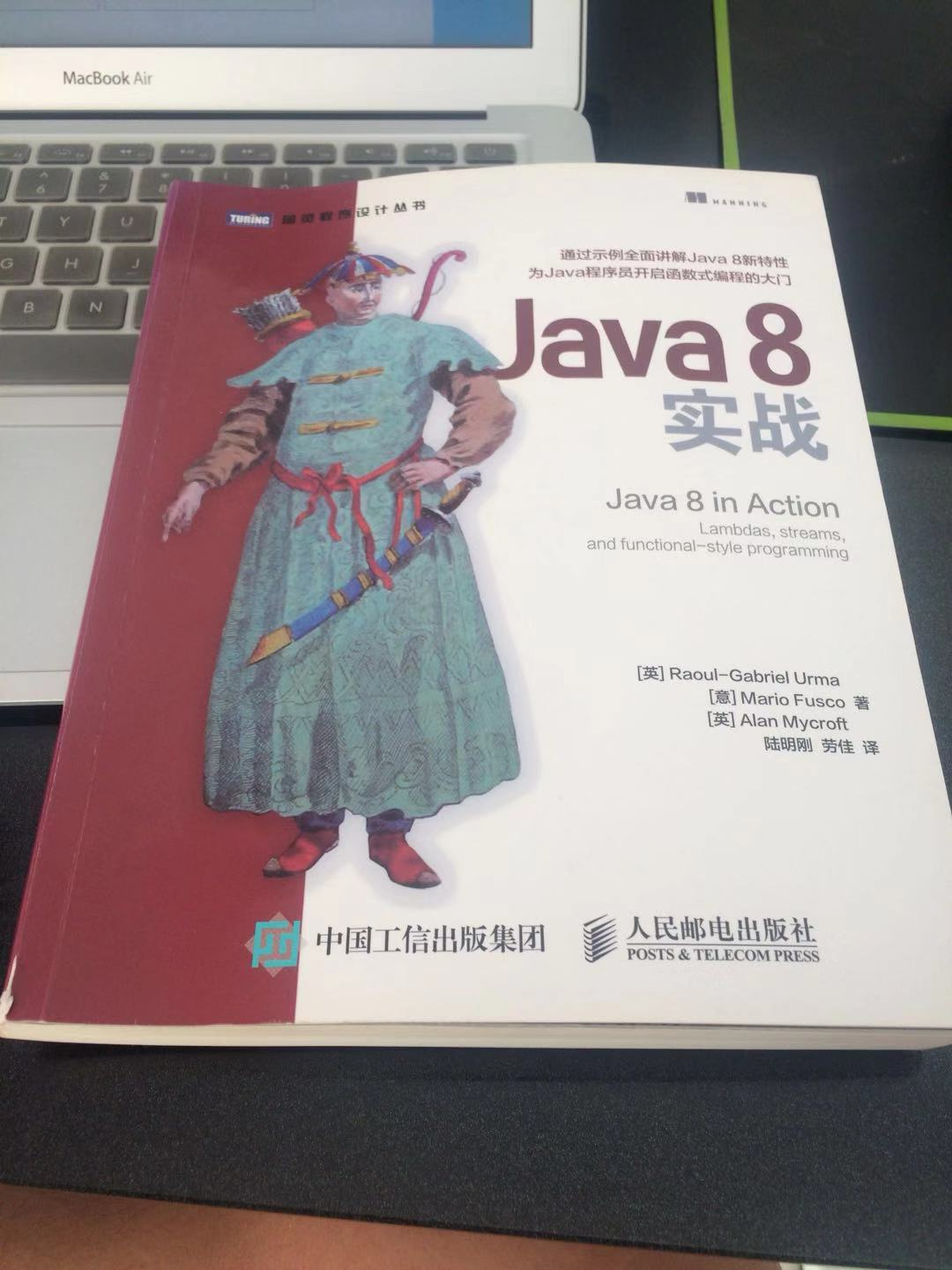•Java 并行计算

# Java 并行计算

## 分支/合并框架

``````import java.util.concurrent.ForkJoinPool;
import java.util.stream.IntStream;
import java.util.stream.LongStream;

public class ForkJoinSumCalculator extends RecursiveTask<Long> {

private final long[] numbers;
private final int start;
private final int end;

public static final long THRESHOLD = 10000;

public ForkJoinSumCalculator(long[] numbers){
this(numbers, 0, numbers.length);
}

public ForkJoinSumCalculator(long[] numbers, int start, int end) {
this.numbers = numbers;
this.start = start;
this.end = end;
}

@Override
protected Long compute() {

int length = end - start;
if(length <= THRESHOLD){
return this.computeSequencially();
}
ForkJoinSumCalculator leftFork = new ForkJoinSumCalculator(numbers, start, start + length / 2);
leftFork.fork();
ForkJoinSumCalculator rightFork = new ForkJoinSumCalculator(numbers, start + length / 2, end);
Long rightResult = rightFork.compute();
Long leftResult = leftFork.join();

return leftResult + rightResult;

}

private long computeSequencially(){
long sum = 0;
for(int i = start; i < end; i++){
sum += numbers[i];
}
return sum;
}

public static long forkJoinSum(long n){
long[] numbers = LongStream.rangeClosed(1, n).toArray();
ForkJoinSumCalculator forkJoinSumCalculator = new ForkJoinSumCalculator(numbers);
return new ForkJoinPool().invoke(forkJoinSumCalculator);
}
}
``````

## 并行流

java8 提供的 Stream 提供了 parallel() 方法可以将默认的顺序流转换为并行流，和 sequential() 方法可以将并行流转为顺序流。但是如果你对要处理的操作是否适合并行计算存有疑问，那么你要小心了，有可能得到的结果与正确结果差十万八千里。并行计算可以和日常生活作类比，对于小任务而言比如一件事情如果一个人就可以很快完成就没有必要雇来十个人做，因为十个人做，任务分配所花的时间有可能很长在这十个人分配任务的时间有可能一个人就已经完成了。所以多人做事情，沟通成本是很高的，但是 stream 并行计算多个线程之间不会沟通和同步，就像十个人同时做事但是他们互相不可交谈，在这样的情况下他们之间不可以共享东西啊，因为一旦他们之间共享一件东西有可能会造成混乱。stream 的并行计算就是如此，对于1.小任务而言没有必要使用并行计算 2.并行计算带来的成本不应该抵消其带来的优势 3.并行计算不应该修改共享变量 4. 该并行计算最好是无序的。

java8 提供了 Spliterator 接口，可以通过实现该接口自定义一个任务的拆分和合并过程以满足自己特殊的并行计算需求。java8 为集合框架提供的所有数据结构提供了默认的 Spliterator 实现。